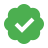# Supervisely/ Confusion MatrixCustom Updated An hour ago Free
Takes 2 (or 1) projects as input and calculates Confusion_Matrix for classes included in classes_mapping

# Confusion matrix.

Classical definition: A confusion matrix is a table that outlines different predictions and test results and contrasts them with real-world values. Confusion matrices are used in statistics, data mining, machine learning models and other artificial intelligence AI applications. A confusion matrix can also be called an error matrix.

But calculating of Confusion matrix for object detection and instance segmentation tasks is less intuitive. First it is necessary to enter a few additional concepts. A key role in calculating metrics for object detection and instance segmentation tasks is played by Intersection over Union(IoU). You can read more about IoU in our other guide(!add link). Here we will briefly describe it.

### Intersection Over Union (IOU)

Intersection Over Union (IOU) is measure based on Jaccard Index that evaluates the overlap between two bounding boxes or instance segments. It requires a ground truth bounding box(instance segment) Bgt and a predicted bounding box(instance segment) Bp.By applying the IOU we can tell if a detection is valid (True Positive) or not (False Positive).
IOU is given by the overlapping area between the predicted instance and the ground truth instance divided by the area of union between them:Some extra basic concepts that we will use:

• True Positive (TP): A correct detection. Detection with IOU ≥ threshold• False Positive (FP): A wrong detection. Detection with IOU < threshold• False Negative (FN): A ground truth not detected• True Negative (TN): Does not apply. It would represent a corrected misdetection. In the object detection task there are many possible bounding boxes that should not be detected within an image. Thus, TN would be all possible bounding boxes that were correctly not detected (so many possible boxes within an image). That's why it is not used by the metrics.

threshold - it is usually set to 0.5, 0.75 or swept from 0.5 to 0.95.

### Confusion matrix

Now we will describe the matrix calculation algorithm for our specific tasks. It consists of following main steps:

1. For each ground-truth box, the algorithm generates the IoU (Intersection over Union) with every detected box. A match is found if both boxes have an IoU greater or equal than set threshold (for example 0.75).

2. The list of matches is pruned to remove duplicates (ground-truth boxes that match with more than one detection box or vice versa). If there are duplicates, the best match (greater IoU) is always selected.

3. The confusion matrix is updated to reflect the resulting matches between ground-truth and detections.

4. Objects that are part of the ground-truth but weren’t detected are counted in the last column of the matrix (in the row corresponding to the ground-truth class). Objects that were detected but aren’t part of the confusion matrix are counted in the last row of the matrix (in the column corresponding to the detected class).

Finally, we get a matrix that has the following structure:

• The horizontal rows represent the target values (what the model should have predicted — the ground-truth)

• The vertical columns represent the predicted values (what the model actually predicted).

• Each row and column correspond to each one of the classes supported by the model.

• The final row and column correspond to the class “nothing” which is used to indicate when an object of a specific class was not detected, or an object that was detected wasn’t part of the ground-truth.## Config example

``````{
"iou": 0.5,
"project_1": "project_1_name",
"project_2": "project_2_name",
"classes_mapping": {
"pr1_cls_name_1": "pr2_cls_name_1",
"pr1_cls_name_2": "pr2_cls_name_2"
}
}
``````

Here:

1. "iou" - Intersection over Union threshold.
2. “project_1” - name of the first project involved in IoU evalutaion.
3. “project_2” - name of the second project involved in IoU evaluation. In case you want to evaluate metric between objects of the same project, you can simply delete this field from config.
4. “classes_mapping” - field matches pairs of objects classes between which IoU will be evaluated.

### Docker Image

`supervisely/metric-confmat:latest`

ID
75279
Version ID
84512
First released
5 months ago
Last updated
An hour ago

s